#### Thank you for registering.

One of our academic counsellors will contact you within 1 working day.

Click to Chat

1800-5470-145

+91 7353221155

CART 0

• 0
MY CART (5)

Use Coupon: CART20 and get 20% off on all online Study Material

ITEM
DETAILS
MRP
DISCOUNT
FINAL PRICE
Total Price: Rs.

There are no items in this cart.
Continue Shopping

# Chapter 7: Trigonometric Ratios of Compound Angles – Exercise 7.1

## Trigonometric Ratios of Compound Angles – Exercise 7.1 – Q.1

We have,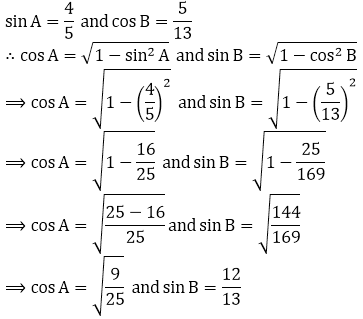Now,

sin(A + B) = sin A cos B + cos A sin BWe have,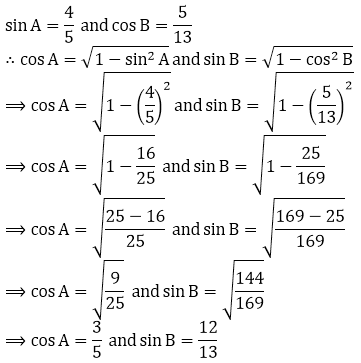Now,

cos(A + B) = cos A cos B - sin A sin BWe have,Now,

Sin (A - B) = sin A cos B - cos A sin B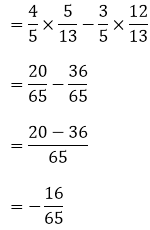We have,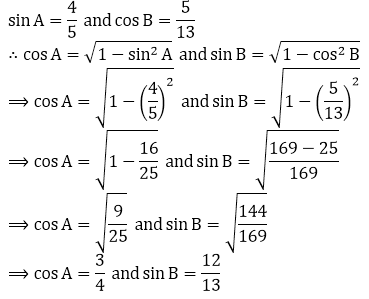Now

cos(A - B) = cos A cos B + sin A sin B## Trigonometric Ratios of Compound Angles – Exercise 7.1 – Q.2

We have,Now,

(i) sin(A + B) = sin A cos B + cos A sin B(ii) cos(A + B) = cos A cos B - sin A sin BWe have,Now,

sin(A+B) = sin A cos B + cos A sin B## Trigonometric Ratios of Compound Angles – Exercise 7.1 – Q.3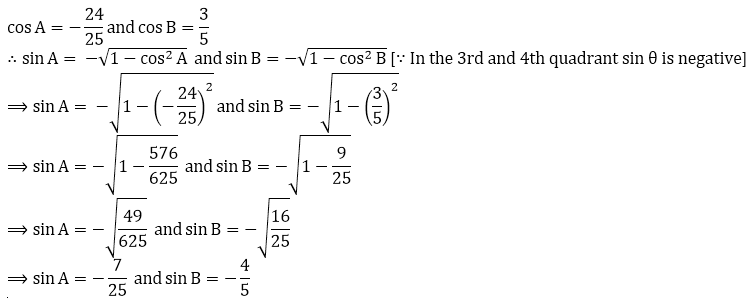Now,

(i) sin(A + B) = sin A cos B + cos A sin B(ii) cos(A+B) = cos A cos B - sin A sin B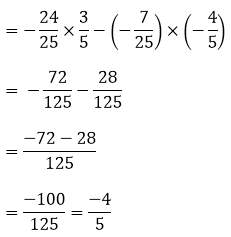## Trigonometric Ratios of Compound Angles – Exercise 7.1 – Q.4

We have,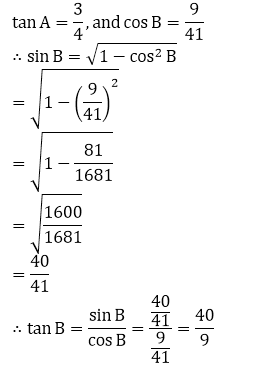Now,